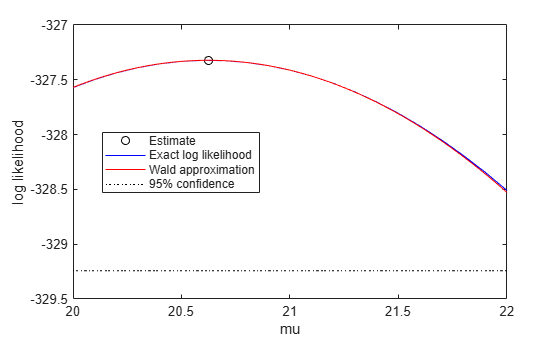proflik

Profile likelihood function for probability distribution

Description

example

[ll,param] = proflik(pd,pnum) returns a vector ll of loglikelihood values and a vector param of corresponding parameter values for the parameter in the position indicated by pnum.

[ll,param] = proflik(pd,pnum,'Display',display) returns the loglikelihood values and corresponding parameter values, and plots the profile likelihood overlaid on an approximation of the loglikelihood.

[ll,param] = proflik(pd,pnum,setparam) returns the loglikelihood values and corresponding parameter values as specified by setparam.

example

[ll,param] = proflik(pd,pnum,setparam,'Display',display) returns the loglikelihood values and corresponding parameter values as specified by setparam, and plots the profile likelihood overlaid on an approximation of the loglikelihood.

example

[ll,param,other] = proflik(___) also returns a matrix other containing the values of the other parameters that maximize the likelihood, using any of the input arguments from the previous syntaxes.

Examples

collapse all

Load the sample data. Create a probability distribution object by fitting a Weibull distribution to the miles per gallon (MPG) data.

pd = fitdist(MPG,'Weibull')
pd =
WeibullDistribution

Weibull distribution
A = 26.5079   [24.8333, 28.2954]
B = 3.27193   [2.79441, 3.83104]

View the parameter names for the distribution.

pd.ParameterNames
ans = 1x2 cell
{'A'}    {'B'}

For the Weibull distribution, A is in position 1, and B is in position 2.

Compute the profile likelihood for B, which is in position pnum = 2.

[ll,param] = proflik(pd,2);

Display the loglikelihood values for the estimated values of B.

[ll',param']
ans = 21×2

-329.9688    2.7132
-329.4312    2.7748
-328.9645    2.8365
-328.5661    2.8981
-328.2340    2.9597
-327.9658    3.0213
-327.7596    3.0830
-327.6135    3.1446
-327.5256    3.2062
-327.4943    3.2678
⋮

These results show that the profile log likelihood is maximized between the estimated B values of 3.2678 and 3.3295, which correspond to loglikelihood values -327.4943 and -327.5178. From the earlier fit, the MLE of B is 3.27193, which is in this interval as expected.

Load the sample data. Create a probability distribution object by fitting a generalized extreme value distribution to the miles per gallon (MPG) data.

pd = fitdist(MPG,'GeneralizedExtremeValue')
pd =
GeneralizedExtremeValueDistribution

Generalized Extreme Value distribution
k = -0.207765   [-0.381674, -0.0338563]
sigma =   7.49674   [6.31755, 8.89604]
mu =   20.6233   [18.8859, 22.3606]

View the parameter names for the distribution.

pd.ParameterNames
ans = 1x3 cell
{'k'}    {'sigma'}    {'mu'}

For the generalized extreme value distribution, k is in position 1, sigma is in position 2, and mu is in position 3.

Compute the profile likelihood for mu, which is in position pnum = 3. Restrict the computation to parameter values from 20 to 22, and display the plot.

[ll,param,other] = proflik(pd,3,20:.1:22,'display','on');The plot shows the estimated value for the parameter mu that maximizes the loglikelihood.

Display the loglikelihood values for the estimated values of mu, and the values of the other distribution parameters that maximize the corresponding loglikelihood.

[ll',param',other]
ans = 21×4

-327.5706   20.0000   -0.1803    7.4087
-327.4971   20.1000   -0.1846    7.4218
-327.4364   20.2000   -0.1890    7.4354
-327.3887   20.3000   -0.1934    7.4493
-327.3538   20.4000   -0.1978    7.4636
-327.3317   20.5000   -0.2023    7.4783
-327.3223   20.6000   -0.2067    7.4932
-327.3257   20.7000   -0.2112    7.5084
-327.3418   20.8000   -0.2156    7.5240
-327.3706   20.9000   -0.2201    7.5399
⋮

The first column contains the log likelihood value that corresponds to the estimate of mu in the second column. The log likelihood is maximized between the parameter values 20.6000 and 20.7000, corresponding to log likelihood values -327.3223 and -327.3257. The third column contains the value of k that maximizes the corresponding log likelihood for mu. The fourth column contains the value of sigma that maximizes the corresponding log likelihood for mu.

Input Arguments

collapse all

Parameter number for which to compute the profile likelihood, specified as a positive integer value corresponding to the position of the desired parameter in the parameter name vector. For example, a Weibull distribution has a parameter name vector {'A','B'}, so specify pnum as 2 to compute the profile likelihood for B.

Data Types: single | double

Parameter value restriction, specified as a scalar value or a vector of such values. If you do not specify setparam, proflik chooses the values for output vector param based on the default confidence interval method for the probability distribution pd. If the parameter can take only restricted values, and if the confidence interval violates that restriction, you can use setparam to specify valid values.

Example: [3,3.5,4]

Display toggle, specified as either 'on' or 'off'. Specify 'on' to display the profile of the exact loglikelihood overlaid on the Wald approximation of the loglikelihood. Specify 'off' to omit the display. The Wald approximation is based on a Taylor series expansion around the estimated parameter value, as a function of the parameter in position pnum or its logarithm. The intersection of the curves with the horizontal dotted line marks the endpoints of 95% confidence intervals.

Output Arguments

collapse all

Loglikelihood values, returned as a vector. The loglikelihood is the value of the likelihood with the parameter in position pnum set to the values in param, maximized over the remaining parameters.

Parameter values corresponding to the loglikelihood values in ll, returned as a vector. If you specify parameter values using setparam, then param is equal to setparam.

Other parameter values that maximize the likelihood, returned as a matrix. Each row of other contains the values for all parameters except the parameter in position pnum.